# DESIGN OF STEEL COMPRESSION MEMBERSReading time: 1 minute

DESIGN OF STEEL COMPRESSION MEMBERS A structural member loaded axially in compression is generally called a compression member. Vertical compression members in buildings are called columns, posts or stanchions. A compression member in roof trusses is called struts and in a crane is called a boom.Columns which are short are subjected to crushing and behave like members under pure compression. Columns which are long tend to buckle out of the plane of the load axis.

#### THEORY OF COLUMNS

Euler’s formula for critical load for a pin-ended column subjected to axial load is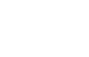Where, L = length of column between the hinged ends, E = modulus of elasticity, and I = moment of inertia of the column section. The column will become unserviceable if the loads are larger than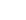. In the Euler equation, it is assumed that stress is proportional to strain, therefore, Critical Stress =Where, A= area of cross-section, and r = radius of gyration about the bending axis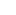= slenderness ratio

#### VARIOUS END CONDITIONS

Columns with length L and effective length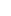are shown in figure below:#### Strength of an Axially Loaded Compression Members

Maximum axial compression load permitted on a compression member,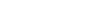Where, P = axial compressive load (N),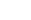= permissible stress in axial compression (MPa) A = effective cross-sectional area of the member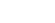Indian Standard IS 800: 1984 It stipulates that the direct stress on the cross-sectional area of axially loaded compression members should not exceed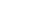nor the permissible stress calculated using Merchant – Rankine formula. Permissible stress in axial compression (MPa):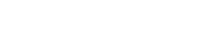Where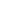= yield stress of steel in MPa= elastic critical stress in compression =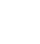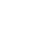= slenderness ratio of the member Where,= effective length of the member r = appropriate radius of gyration of the member E = modulus of elasticity = 200000 MPa, and n = a factor assumed as 1.4 EFFECTIVE LENGTH OF COMPRESSION MEMBER Table below gives the values of effective length recommended by the Indian Standard, IS 800. The actual length L of the compression member should be taken as the length from centre-to-centre of intersection of supporting members or the cantilevered length in the case of free standing struts. Table: Equivalent length for various end conditions
 Type Effective length of member l 1 Effectively held in position and restrained in direction at both ends. 0.67 L 2 Effectively held in position at both ends restrained in direction at one end. 0.85 L 3 Effectively held in position at both ends but not restrained in direction. L 4 Effectively held in position and restrained in direction at one end and at the other end effectively restrained in direction but not held in position. L 5 Effectively held in position and restrained in direction at one end and the other end partially restrained in direction but not held in position. 1.5 L 6 Effectively held in position and restrained in direction at one end but not held in position or restrained in direction at the other end. 2.0 L
Note:
1. L is the unsupported length of compression member.
2. For battened struts, the effective length should be increased by 10%.

#### MAXIMUM SLENDERNESS RATIO:

According to Indian Standard IS 800, the slenderness ratio should not exceed the values given in the table below:
 No. Type of Member Slenderness ratio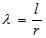1 A member carrying compressive loads resulting from dead and superimposed loads. 180 2 A member subjected to compressive loads resulting from wind/earthquake forces provided the deformation of such members does not adversely affect the stress in any part of the structure. 250 3 A member normally carrying tension but subjected to reversal of stress due to wind or earthquake forces. 350 4 Tension member (other than pre-tensioned member) 400

#### ANGLE STRUTS

Single angle discontinuous struts connected by a single rivet or bolt may be designed for axial load only provided the compressive stress does not exceed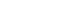. The value ofcan be determined on the basis that the effective length l of the strut is from centre-to-centre of inter-section at each end and r is the minimum radius of gyration. In no case, the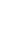ratio for single angle struts should exceed 180. If a single discontinuous strut is connected by a weld or by two or more rivets or bolts in line along the angle at each end, it may be designed for axial load only provided the compression stress does not exceedarrived at on the basis that l is taken as 0.85 times the length of the strut, centre to centre at each end and r is the minimum radius of gyration. For double angle struts which are discontinuous, back to back connected to both sides of the gusset or section by not less than two bolts or rivets in line along the angles at each end or by equivalent welding, the load may be regarded as applied axially. The effective length l in the plane of end gusset could be taken between 0.7 and 0.85 times the distance between the intersection depending on the restraint provided, the plane perpendicular to that of the end gusset, the effective length should be taken as equal to the distance between centers of intersections. The calculated average compressive stress should not exceed values ofobtained for the appropriate slenderness ratios. The angles should be connected together with tack rivets or welds at intervals along their lengths.

#### COMPRESSION MEMBERS COMPOSED OF BACK-TO-BACK COMPONENTS

A compression member composed of two angles, channels or tees, back to back, in contact or separated by a small distance should be connected together by riveting, bolting or welding so that the slenderness ratio of each member between the connections is not greater than 40 nor greater than 0.60 times the most unfavourable slenderness ratio of the strut as a whole. In no case, the spacing of tacking rivets in a line exceed 600mm for such members. For other types of built-up compression members, where cover plates are used, the pitch of tacking rivets should not exceed 32t or 300mm, whichever is less, where t is the thickness of the thinner outside plate. Where plates are exposed to bad weather conditions, the pitch should not exceed 16 t or 200mm whichever is less. The rivets, welds and bolts in these connections should be sufficient to carry the shear force and bending moments, if any, specified for battened struts. The diameter of the connecting rivets should not be less than the minimum diameter given in the table below:
 Thickness of member Minimum diameter of rivets Upto 10mm 16mm Over 10mm upto 16mm 20mm Over 16mm 22 mm

Solid packing or washers should be used for riveting, bolting, where the members are separated back to back.

The end struts should be connected together with not less than two rivets or bolts or their equivalent in welding and there should be not less than two additional connections spaced equidistant in the length of the strut.

A minimum of two rivet or bolts should be used in each connection, one on line of each gauge mark, where the legs of the connected angles or tables of the connected tees are 125mm wide or over, or where the webs of channel are 150mm wide or over.

#### LACINGS AND BATTENS FOR BUILT-UP COMPRESSION MEMBERS

As per Indian Standard, IS 800-1984, the following specifications are used for the design of lacing and batten plates.

In a built-up section, the different components are connected together so that they act as a single column. Lacing is generally preferred in case of eccentric loads. Battening is normally used for axially loaded columns and in sections where the components are not far apart. Flat bars are generally used for lacing. Angles, channels and tubular sections are also used for lacing of very heavily columns. Plates are used for battens.

LacingsA lacing system should generally conform to the following requirements:

1. The compression member comprising two main components laced and tied should, where practicable, have a radius of gyration about the axis perpendicular to the plane of lacing not less than the radius of gyration at right angles to that axis.
2. The lacing system should not be varied throughout the length of the strut as far as practicable.
3. Cross (except tie plates) should not be provided along the length of the column with lacing system, unless all forces resulting from deformation of column members are calculated and provided for in the lacing and its fastening.
4. The single-laced systems on opposite sides of the main components should preferably be in the same direction so that one system is the shadow of the other.
5. Laced compression members should be provided with tie plates at the ends of the lacing system and at points where the lacing system are interrupted. The tie plates should be designed by the same method as followed for battens.

#### GUIDELINES FOR THE DESIGN OF LACING SYSTEM

1. The angle of inclination of the lacing with the longitudinal axis of the column should be between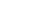to.
2. The slenderness ratio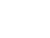of the lacing bars should not exceed 145.
3. The effective length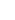of the lacing bar should be according to the table given below:
 No. Type of lacing Effective length,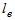1 Single lacing, riveted at ends Length between inner end rivets on lacing bar. 2 Double lacing, riveted at ends 0.7 times the length between end rivets on lacing bars. 3 Welded lacing 0.7 times the distance between inner ends or effective lengths of welds at ends.

1. For riveted or welded lacing system,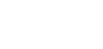or 0.7 times maximum slenderness ratio of the compression member as a whole, whichever is less.
Here, L = distance between the centers of connections of the lattice bars, and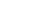= the minimum radius of gyration of the components of the compression member.1. Minimum width of lacing bars in riveted connection should be according to the Table given below:
 Nominal rivet diameter (mm) 22 20 18 16 Width of lacing bars (mm) 65 60 55 50
1. Minimum thickness of lacing bars:, for single lacing;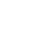, for double lacing Where= length between inner end rivets.
1. The lacing of compression members should be designed to resist a transverse shear, V=2.5 percent of the axial force in the member. The shear is divided equally among all transverse lacing systems in parallel planes. The lacing system should also be designed to resist additional shear due to bending if the compression member carries bending due to eccentric load, applied end moments, and / or lateral loading.
2. The riveted connections may be made in two ways, as shown in the figure (a) and (b).WELDED CONNECTION Lap Joint – Overlap should not be less than ¼ times thickness of bar or member, whichever is less. Butt Joint – Full penetration butt weld or fillet weld on each side, lacing bar should be placed opposite to flange or stiffening component of main member.

#### BATTENS

Compression members composed of two main components battened should preferably have these components of the same cross-section and symmetrically disposed about their X – X axis. The battens should be placed opposite to each-other at each end of the member and at points where the member is stayed in its length, and should as far as practicable, be spaced and proportioned uniformly throughout. The effective length of columns should be increased by 10 percent.

#### Design Details of Battens

1. Spacing of batten C, from centre-to-centre of end fastening should be such that the slenderness ratio of the lesser main component,or 0.7 times the slenderness ratio of the compression member as a whole about X – X axis (parallel to battens) whichever is less.
2. Effective depth of battens, d shall be taken as distance between end rivets or end welds.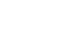for intermediate batten d > a, for end batten d > 2b , for any batten where d = effective depth of batten a = centroidal distance of members b = width of members in the plane of battens.
1. Thickness of battens,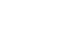Where,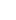= distance between the innermost connecting line of rivets or welds.

#### DESIGN OF BATTENS

Battens should be designed to carry bending moment and shear arising from a transverse shear,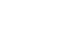Where P = total axial load in the compression member. Transverse shear V is divided equally between the parallel planes of battens. Battens and their connections to main components resist simultaneously a longitudinal shear.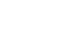and , moment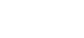due to transverse shear V. where, C = spacing of battens N= number of parallel planes of battens S= minimum transverse distance between centroid of rivet group or welding. The end connections should also be designed to resist the longitudinal shear force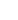and the moment M.

For welded connection

1. Lap < 4t
2. Total length of weld at edge of batten < D/2
a + b+ c < D/2 let t = thickness of batten Length of continuous weld at each edge of batten < 1/3 of total length required. Return weld along transverse axis of the column < 4t Where, t and D are the thickness and overall depth of battens, respectively.